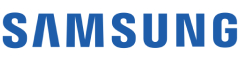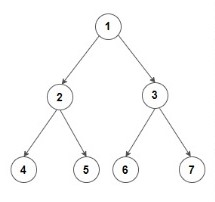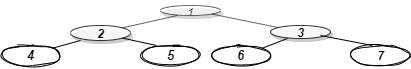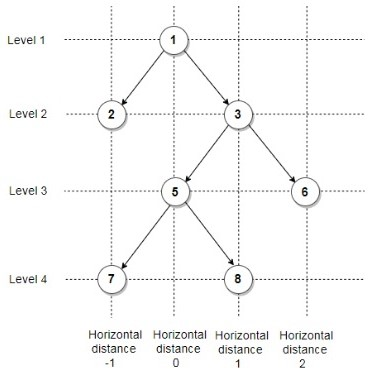New update is available. Click here to update.

# Bottom View Of Binary Tree

Contributed by
Dhruv Sharma
Medium0/80
10 mins
90 %72 upvotes+14 more

## Problem Statement

#### Return the bottom view of the binary tree.

##### Note :
``````1. A node will be in the bottom-view if it is the bottom-most node at its horizontal distance from the root.

2. The horizontal distance of the root from itself is 0. The horizontal distance of the right child of the root node is 1 and the horizontal distance of the left child of the root node is -1.

3. The horizontal distance of node 'n' from root = horizontal distance of its parent from root + 1, if node 'n' is the right child of its parent.

4. The horizontal distance of node 'n' from root = horizontal distance of its parent from the root - 1, if node 'n' is the left child of its parent.

5. If more than one node is at the same horizontal distance and is the bottom-most node for that horizontal distance, including the one which is more towards the right.
``````

##### Example:
``````Input: Consider the given Binary Tree:
````````````Output: 4 2 6 3 7

Explanation:
Below is the bottom view of the binary tree.
````````````1 is the root node, so its horizontal distance = 0.
Since 2 lies to the left of 0, its horizontal distance = 0-1= -1
3 lies to the right of 0, its horizontal distance = 0+1 = 1
Similarly, horizontal distance of 4 = Horizontal distance of 2 - 1= -1-1=-2
Horizontal distance of 5 = Horizontal distance of 2 + 1=  -1+1 = 0
Horizontal distance of 6 = 1-1 =0
Horizontal distance of 7 = 1+1 = 2

The bottom-most node at a horizontal distance of -2 is 4.
The bottom-most node at a horizontal distance of -1 is 2.
The bottom-most node at a horizontal distance of 0 is 5 and 6. However, 6 is more towards the right, so 6 is included.
The bottom-most node at a horizontal distance of 1 is 3.
The bottom-most node at a horizontal distance of 2 is 7.

Hence, the bottom view would be 4 2 6 3 7
``````

Detailed explanation ( Input/output format, Notes, Images )

#### Sample input 1 :

``````1 2 3 -1 -1 5 6 7 8 -1 -1 -1 -1 -1 -1
``````

#### Sample output 1 :

``````7 5 8 6
``````

#### Explanation of sample input 1 :

``````Test case 1:
````````````As shown in the above figure,

1 is the root node, so its horizontal distance = 0.
Since 2 lies to the left of 0, its horizontal distance = 0-1= -1
3 lies to the right of 0, its horizontal distance = 0+1 = 1
Similarly, horizontal distance of 5 = Horizontal distance of 3 - 1= 1-1= 0
Horizontal distance of 6 = Horizontal distance of 3 + 1=  1+1 = 2
Horizontal distance of 7 = 0-1 =-1
Horizontal distance of 8 = 0+1 = 1

The bottom-most node at a horizontal distance of -1 is 7.
The bottom-most node at a horizontal distance of 0 is 5.
The bottom-most node at a horizontal distance of 1 is 8.
The bottom-most node at a horizontal distance of 2 is 6.

Hence, the bottom view would be 7 5 8 6.
``````

#### Sample input 2 :

``````1 2 3 4 -1 6 7 -1 -1 -1 8 -1 -1 -1 -1
``````

#### Sample output 2 :

``````4 2 6 8 7
``````

##### Expected Time Complexity:
``````Try to do this in O(n*log(n)).
``````

##### Constraints:
``````1 <= n <= 10000

Where 'n' is the total number of nodes in the binary tree.

Time Limit: 1 sec
``````AutocompleteConsole